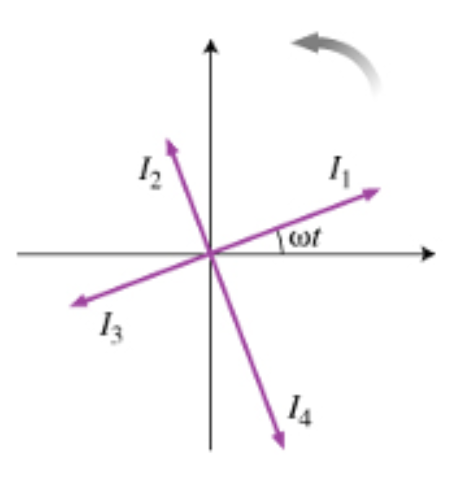# Problem: Let us assume that a certain quantity I(t) changes over time as I(t) = I0cos(ωt). A phasor is a vector whose length represents the amplitude I0 (see the diagram (Figure 1) ). This vector is assumed to rotate counterclockwise with angular frequency ω, that way, the horizontal component of the vector represents the actual value i(t) at any given moment. In this problem, you will answer some basic questions about phasors and prepare to use them in the analysis of various AC circuits.At the moment t depicted in the diagram, which of the following statements is true? A) I2 leads I1 by πB) I1 leads I2 by πC) I2 leads I1 by π/2D) I1 leads I2 by π/2

###### FREE Expert Solution

I2 is ahead of I1 by π/2 (or 90°), since the rotation of the phasors is counterclockwise

93% (306 ratings)###### Problem Details

Let us assume that a certain quantity I(t) changes over time as I(t) = I0cos(ωt). A phasor is a vector whose length represents the amplitude I0 (see the diagram (Figure 1) ). This vector is assumed to rotate counterclockwise with angular frequency ω, that way, the horizontal component of the vector represents the actual value i(t) at any given moment. In this problem, you will answer some basic questions about phasors and prepare to use them in the analysis of various AC circuits.At the moment t depicted in the diagram, which of the following statements is true?

A) I2 leads I1 by π

B) I1 leads I2 by π

C) I2 leads I1 by π/2

D) I1 leads I2 by π/2